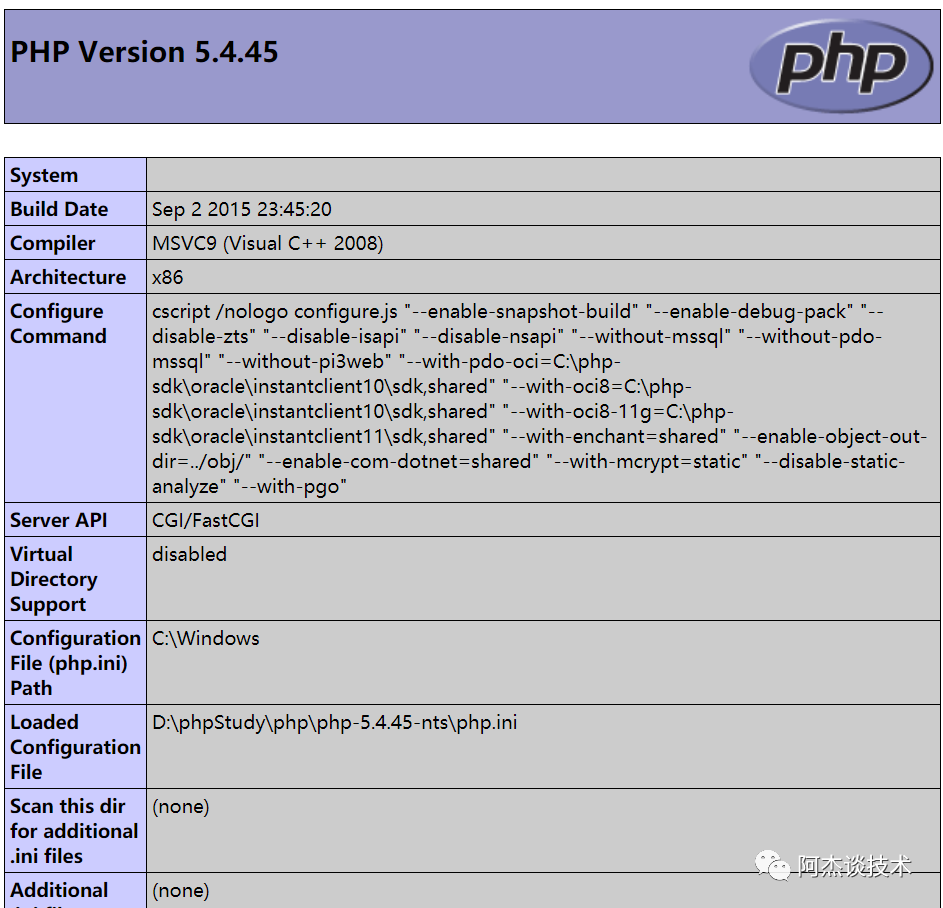# PHP基础入门(一)

+关注继续查看

## PHP 基础语法

### php函数(危险指数五颗星)

<?php
phpinfo();
?>### 变量

#### 内部变量

<?php
$name='阿杰';$age=20;
echo '你的名字是'.$name; echo '你的年龄是'.$age;
?>

#### 外部变量

<html>
<body>
<!-- 这一行method 对应的值改为post --->
<form action="req.php" method="post">
<input type="submit" name="提交"/>
</form>
</body>
</html>

PHP代码调用以上HTML代码中的外部变量方法如下所示：

<?php
$u =$_POST['username'];
echo $u.'<br />';$passwd = $_POST['pwd']; echo$passwd.'<br />';
?>

1 $_COOKIE 得到会话控制中的cookie传值信息 2$_SESSION 得到会话控制中的session传值信息

3 $_FILES 得到文件上传的结果 4$_GET 得到get传值的结果

5 $_POST 得到post传值的结果 6$_REQUEST 可以得到get的传值结果，也能得到POST传值的结果

$_SERVER[“REQUEST_METHOD”] 请求当前PHP页面的方法$_SERVER[“REQUEST_URI”] 请求当前PHP页面的URI

$_SERVER[“SERVER_SOFTWARE”] 用的是哪一种网站服务程序$_SERVER[“REMOTE_ADDR”] 客户端的IP地址

$_SERVER[“SERVER_ADDR”] 当前服务器的IP地址$_SERVER[“SCRIPT_FILENAME”] 当前请求文件的路径信息

$_SERVER[“HTTP_USER_AGENT”] 当前访问这个网址的电脑和浏览器的情况$_SERVER[“HTTP_REFERER”] 上级来源（用户从哪个地址进入当前网页）

$_SERVER[“REQUEST_TIME”] 当前的访问时间信息 ### 常量 <?php define('name','阿杰'); echo '你的名字'.name; ?> 以下是一些内置的常量 常量名 变量解释说明 LINE 当前所在的行 FILE 当前文件在服务器的路径 FUNCTION 当前函数名称 CLASS 当前类名 METHOD 当前成员方法名 PHP_OS PHP运行的操作系统 PHP_VERSION 当前PHP的版本 TRAIT Trait的名字php5.4新加常量 DIR 文件所在的目录信息 NAMESPACE 当前命名空间的名称（区分大小写） ### 注释 // 单行注释 # 单行注释 /* 多行注释 */ ### 数据类型 php中有八种数据类型 四种标量数据类型 boolean(布尔类型) integer(整形) float/double(浮点型) string(字符串型) 两种复合数据类型 array(数组) object(对象) 两种特殊类型 resource(资源类型) null(无/空白类型) 数据类型 数据类型描述 boolean（布尔类型） 布尔型是简单的数据类型，只有两个值false（假）和true（真） string（字符串型） 字符串就是连续的字符序列，如echo“string”； integer（整形） 整形数据类型只能包含整数，这些数据类型可以是负数或者整数 float/double（浮点型） 浮点类型用于存储数字，和整形不同的是浮点型可以有小数点 array（数组类型） 一组相同类型的集合 object（对象类型） 对象是一个实例，使用new命令创建一个对象 resource（资源型） 资源是一种特殊的变量，保存在外部资源的一个应用，资源是通过函数来进行建立的 null（空白） 特殊的值，表示变量没有值，任何变量的初始值都是null #### 整形 <?php // 进制的取值范围是0-7，即0、1、2、3、4、5、6、7$i = 01234;
// 10进制
$i = 100; // 16进制取值范围是0-f，即0、1、2、3、4、5、6、7、8、9、a、b、c、d、e、f$i = 0x6ff;
?>

#### 布尔

<?php
$blue=true;$red=false;
?>

#### 字符串

<?php
// 单引号
$name = '阿杰'; // 双引号$name = "阿杰";
// 多行字符
$line = <<<ABC 文字 文字 文字 ABC; echo$line;
?>

#### 浮点

<?php
// 普通声明
$f = 124.34525; // 科学声明$f = 0.423534;
var_dump($f) ?> ### 判断数据类型 gettype（传入一个变量）能获得变量的类型 var_dump（传入一个变量）输出变量类型和值 <?php$f = 88.8;
$type=gettype($f);
$s = '男人习惯了不说 忍不住了就抽根烟吧';$type = var_dump($s); ?> 判断数据类型方法： 判断函数 函数判断描述 is_int 判断是否为整数数据类型 is_bool 判断是否为布尔数据类型 is_float 判断是否是浮点数据类型 is_string 判断是否是字符串数据类型 is_array 判断是否是数组数据类型 is_object 判断是否是对象数据类型 is_null 判断数据信息是否为空 is_resource 判断是否为资源数据类型 is_scalar 判断是否为标量 is_numeric 判断是否为数值类型 is_callable 判断是否为函数 <?php //is_*系列函数有个特点，就是如果是这个类型的返回的值为真，不是这个数据类型就返回的是假 //声明类型为假$fo = false;
if (is_bool($fo)) { echo '执行真区间'; }else{ echo '执行假区间'; } echo "<br />"; //检查未声明的变量$p是否为空，为空就执行真区间
if(is_null($p)) { echo '执行真区间'; }else{ echo '执行假区间'; } echo "<br />"; //字符串类型的数值，检查执行的真还是假$str = '18.8';
if(is_numeric($str)) { echo '执行真区间'; }else{ echo '执行假区间'; } echo "<br />"; //把sca的值换为整数 浮点 布尔和字符串试试$sca = true;
if($i){ echo '666'; } else{ echo '999'; } ?> ### 算数运算方法 符号信息 说明 举例说明 + 表示相加符号$x+$y - 表示相减符号$x-$y * 表示相乘符号$x*$y / 表示相除符号$x/$y % 表示取余或取模符号$x%$y <?php$x = 6;
$y = 7; // 6+7=13 echo$x +$y; ?> 符号信息 说明 举例说明$x++ 表示先赋值后累加运算

$x-- 表示先赋值后求差运算 ++$x 表示先累加运算后赋值 $x*$y

--$x 表示先求差运算后赋值$x/$y <?php$x=5;
//先赋值后做加法运算，即将$x的值赋值为$y.$x的值为5，所以将$x的值赋值为$y.$y也是5
$y=$x++;
//$x的结果输出为6，因为赋值给$y后，$x自己又把自己进行了+1操作。所以，$x结果为6
echo "$x$y";
?>

> 表示大于符号

< 表示小于符号

>= 表示大于等于符号

<= 表示小于等于符号

== 表示等于符号

!= 表示不等于符号

=== 表示全等（判断类型等于）

!== 表示全不等（判断类型不等于）

<?php
$x=5;$y=6;
//因为5大于6不成立，所以为错的，即为false执行了假区间
if($x >$y){
echo '变量x大于变量y 成立';
}else{
echo '变量x大于变量y 不成立';
}
?>

$y=false; //逻辑与（并且），要求两个都为true才能执行真区间，所以代码中执行假区间 if($x && $y) { echo '执行了真区间'; }else{ echo '执行了假区间'; } ### 程序位运算 符号信息 说明 举例说明 按位与And 将把$a和$b中都为1的位设为1$a & $b 按位或Or 将把$a和$b中任何一个为1的位设为1$a | $b 按位异或Xor 将把$a和$b中一个为1另一个为0的位设置为1$a ^$b 按位取反Not 将把$a中为0的位设为1,反之亦然 ~$a 左移 将把$a中的位向左移$b次(每一次移动都表示乘以2)$a<$b 右移 将把$a中的位向左右$b次(每一次移动都表示乘以2)$a>$b <?php //$x二进制为:
$x=5; //$y二进制为:
$y=8; //结果为13 echo$x ^ $y; ?> ### 三元运算符合其它运算符 符号信息 说明$x?真代码段:假代码段 判断是否为真假？真情况：假情况

反引号 反引号中间插入代码，执行系统命令，等价于shell_exec函数

@ 表示抑制错误，把这一行的错误不让它显示出来了，效率低不建议用

=> 数组下标访问符

-> 对象访问符

instanceof 判断某个对象是否来自某个类，如果是的返回true，如果不是返回false

<?php
// 设x为真 如果x为真输入y 否则输出6
$x=true;$x?$y=5:$y=6;
//输出5
echo $y; ?> <?php echo '<pre>'; // 反引号里执行cmd命令 echo systeminfo; echo '</pre>'; ?> ### if多条件结构流程 <?php If(判断语句1){ 执行语句体1 }elseif（判断语句2）{ 执行语句体2 } elseif（判断语句n）{ 执行语句体n }else{ 最后的else语句可选 } ?> <?php$week=date("4");
//判断星期小于6，则输出：还没到周末，继续上班....
if($week<"6"){ echo "还没到周末，继续上班...."; } ?> <?php //定义一个随机变量，抵达时间，随机0点至23点$dida = rand(0,23);
echo "当前时间为 $dida 点\n"; if($dida > 6 && $dida < 10 ){ echo '酒店去泡澡'; }elseif ($dida > 10 && $dida < 14){ echo '吃神户牛肉'; }elseif($dida >= 19 && $dida < 22){ echo '找个朋友聊聊内心的寂寞'; }elseif($dida > 22 && $dida <=23){ echo '酒店去泡澡'; }elseif($dida >=1 && $dida <3){ echo '夜店参加舞会'; }else{ echo '睡觉或者工作中'; } ?> ### if条件嵌套语句 <?php if(判断1){ if(判断2){ 代码段1; }else{ 代码段2; } }else{ if(判断3){ 代码段3; }else{ 代码段4; } } ?> <?php //0表示工作秘书,1表示生活秘书 //用代码模拟随机产生当前的行程安排是生活秘书负责还是工作秘书负责$mishu=rand(0,1);
if($mishu){ //下雨和不下雨的状态,随机产生;下雨状态为1,不下雨状态为0$xiayu=rand(0,1);
if($xiayu){ //是否购买雨伞;购买了雨伞为1,未购买雨伞为0$you=rand(0,1);
if($you){ echo '下雨天,已经购买雨伞'; }else{ echo '下雨天,需要购买雨伞'; } }else{ //是否购买了防晒霜,购买了为1,未购买为0$you=rand(0,1);
if($you){ echo '未下雨,已经购买了防晒霜'; }else{ echo '未下雨,需要购买防晒霜'; } } }else{ //是否准备好了会议议程,准备好了为1,未准备好为0$yicheng=rand(0,1);
switch($tool){ case 1: echo '司机开车'; break; case 2: echo '民航'; break; case 3: echo '自己家的专机'; break; case 4: echo '火车动车'; break; case 5: echo '骑马'; break; case 6: echo '游轮'; break; } ### while循环语句说明 <?php //定义需要往返的次数,老外喜欢从0开始计数,所以系统也是从0开始$count=0;
//while后面接布尔值判断,为真执行,为假停止
//$count小于100的时候执行,也就是$count为0至99的时候执行.
//如果$count不小于100了,循环停止执行后续的代码 //循环开始处 while($count < 100){
echo "这是王总第 $count 次出差 <br />"; //每次执行让$count+1,这样的话,就不会产生$count永远小于100的情况了$count++;
}
//循环结束
?>
do..while与while区别
do..while与while的语法结构基本一样,也是一个布尔型循环,功能也基本一样.

do{
//代码框
}while(判断)
do…while与while区别是,它们的值得检查时机不同.
do…while不论while判断是否成立,先执行一次代码框循环语句,保证会执行一次(表达式的真值在每次循环结束后检查)

<?php
$i=0; do { echo$i;
}while($i >0); ?> ### for循环语句说明 for(表达式1;表达式2;表达式3) 需要执行的代码段; } <?php for($i=1;$i<=10;$i++)
echo ‘分手后第’.$i.’年, 我全都忘了你的样子<br />’; }?> #### for循环遍历索引数组 <?php //声明一个数组，值为1到10$num = array(1,2,3,4,5,6,7,8,9,10);
//按照索引数组的特点，下标从0开始。所以1的下标为0，10的下标为9
echo $num.'<br />'; echo$num.'<br />';
//我们可以得到数组中元素的总个数,为10
echo count($num) .'<br />'; //遍历这个索引数组的话，我们就可以定义一个变量为$i
//$i 的值为0，从0开始 //可以设定一个循环条件为：$i 在下标的(9)最大值之内循环
for($i = 0 ;$i < count($num) ;$i++){
echo $num.'<br />'; ?> #### 常用操作数组函数 函数信息 说明 array_shift 弹出数组中的第一个元素 array_unshift 在数组的开始处压入元素 array_push 向数组的末尾处压入元素 array_pop 弹出数组末尾的最后一个元素 current 读出指针当前位置的值 key 读出指针当前位置的键 next 指针向下移 prev 向上移 reset 指针到开始处 end 指针到结束处 这些函数，也是面试中基础面试中最爱问到的问题。 序号 函数信息 说明 实例 1 array_combine() 生成一个数组,用一个数组的值作为键名,另一个数组值作为值 2 range() 创建并返回一个包含指定范围的元素的数组。 3 compact() 创建一个由参数所带变量组成的数组 4 array_fill() 用给定的值生成数组 5 array_chunk() 把一个数组分割为新的数组块 6 array_merge() 把两个或多个数组合并为一个数组 array_slice() 在数组中根据条件取出一段值，并返回 array_diff() 返回两个数组的差集数组 array_search() 在数组中搜索给定的值，如果成功则返回相应的键名 array_splice() 把数组中的一部分去掉并用其它值取代 array_sum() 计算数组中所有值的和 in_array() 检查数组中是否存在某个值 array_key_exists() 检查给定的键名或索引是否存在于数组中 shuffle() 将数组打乱,保留键值 count() 计算数组中的单元数目或对象中的属性个数 array_flip() 返回一个键值反转后的数组 array_keys() 返回数组所有的键,组成一个数组 array_values() 返回数组中所有值，组成一个数组 array_reverse() 返回一个元素顺序相反的数组 array_count_values() 统计数组中所有的值出现的次数 array_rand() 从数组中随机抽取一个或多个元素,注意是键名 array_unique() 删除重复值，返回剩余数组 sort() 按升序对给定数组的值排序,不保留键名 rsort() 对数组逆向排序,不保留键名 asort() 对数组排序,保持索引关系 arsort() 对数组逆向排序,保持索引关系 ksort() 按键名对数组排序 krsort() 将数组按照键逆向排序 natsort() 用自然顺序算法对数组中的元素排序 natcasesort() 自然排序,不区分大小写 array_filter() 去掉数组中的空元素或者预定元素 extract 将键变为变量名，将值变为变量值 功能：弹出数组中的第一个元素 <?php$mingren = array("邓超", "黄晓明", "宁泽涛", "钟汉良");
$dc = array_shift($mingren);
echo $dc .'<br />'; print_r($mingren);
?>

### goto循环语句

<?php
goto wan;
echo '天王盖地虎';
wan:
echo '小鸡炖蘑菇';
?>
<?php
for ($i=0;$i<100;$i++){ echo '第'.$i.'每周往返北京大连<br />';
if(\$i == 17){
goto end;
}
}
end:
echo '集团公司要求停止此项';
?>

|
2月前
|

PHP环境搭建（安装MySQL）
PHP环境搭建（安装MySQL）
25 0
|
3月前
|

PHP 原生操作 Mysql 增删改查案例
PHP 原生操作 Mysql 增删改查案例
63 0
|
3月前
|

PHP 原生操作 Mysql
PHP 原生操作 Mysql
55 0
|
3月前
|

PHP 原生连接 Mysql
PHP 原生连接 Mysql
72 0
|
3月前
|

PHP MySql 安装与连接
PHP MySql 安装与连接
92 0
|
4月前
|

PHP的学习与MYSQL高级 2
PHP的学习与MYSQL高级
48 0
|
4月前
|

PHP的学习与MYSQL高级 1
PHP的学习与MYSQL高级
49 0
|
7月前
|

PHP基础入门(二)
PHP基础
29 0
|